# Team:Tsinghua-A/Project/Scalability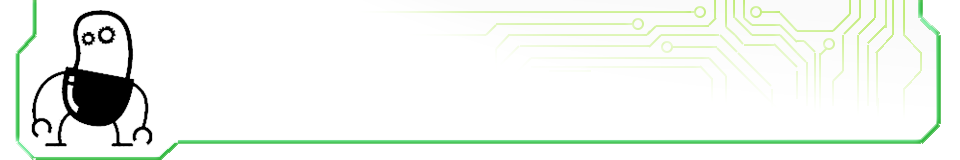## Tsinghua-A::Project::Scalability## Scalability

We can transform AND gate into OR gate through a flip of the sequence between opposite loxP sites. Moreover, based on a similar idea, our system shows a strong scalability in further design.
AND / OR Transformation is a typical pattern of two-input programmable logic devices. There are 16 kinds of two-input logical relations in all, as the table below shows.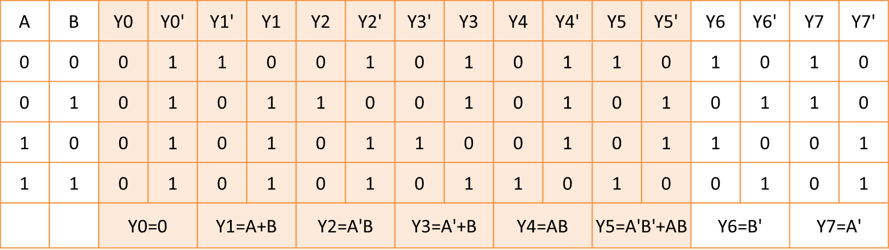Yi and Yi’ (i=0⋯7) stand for 8 logical relations seperately. For example, y1 stands for OR gate and y1’ stands for NOR gate.
Pay attentions to the truth table. Y0(Y0’) shows that the output is always 0(1),which stands for a zero-input logical relation. Y6(Y6’) and Y7(Y7’) stand for one-input logical relation, which can be realized easily. Therefore, only the other 10 kinds of logical relations are real two-input logic which we really care about. Thus, we build a flip system with three modules.

Flip Module 1: It’s same with our core module, which can help us choose whether we want a AND logic or an OR logic. For example, we can transform AND gate(Y4) to OR gate(Y1) by this module.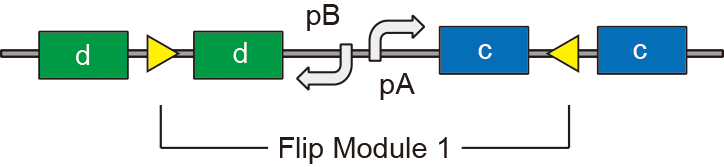Flip Module 2: With the help of this module, we can transform A to A’ in our logic. For example, as the figure below shows, we use Y as our output, now Y = A’B(Y2), and we will get AB if we flip the Flip Module 2, then Y will be AB(Y4).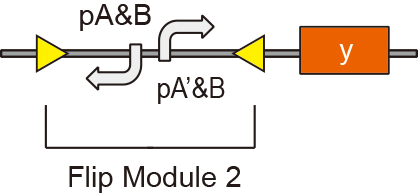Flip Module 3: Using this module, we will be able to get XNOR gate(Y5) because it will help us start another circuit, as the figure below shows. For example, once the Flip Module 3 is activated, the expression of Y will be under the control of the promoter in this sequence. Combining it with the sequence above, Y will be expressed once A’B’ or AB is true.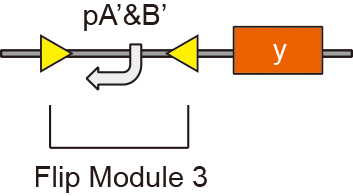Thus, we have realized the transformation of all these 10 kinds of logic relations using this system. The figure below is the whole system.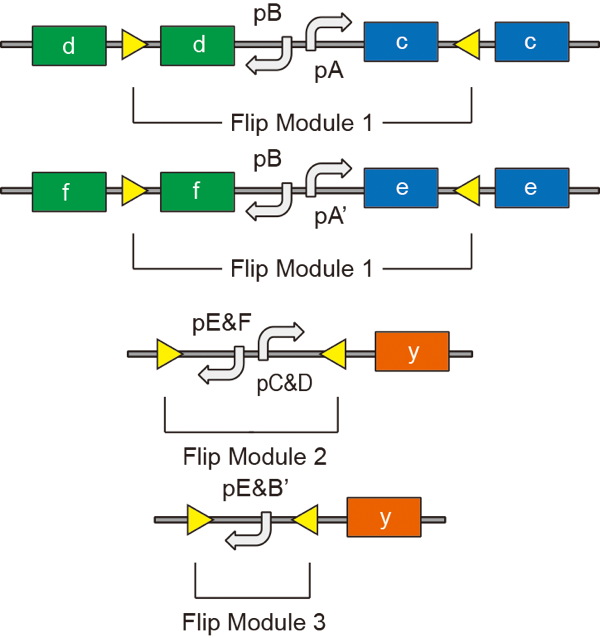When deal with N-input system, we use a more clear and modularized design. All two-input logical relations can be divided into AB, AB’, A’B, A’B’, so we have the equation Y = a*AB + b*AB' + c*A’B + d*A’B’(a, b, c, d=0 or 1)
Therefore, there is an easy thought building module as follows:

The output of AB, A’B, AB’ and A’B’ depends on these four flip module. For example, if Flip Module 1 is flipped, AB can’t influence the expression of Y.

Although we can express all relations in this way, it will cost one more flip module. In addition, if we want to express logical relations with N-input, we will have to build 2^n flip modules. Obviously, it’s difficult to put into application if we need to use more inputs. Comparing with this method, with less flip modules, the previous design is more expected.

From the discussion above, we can find that all two-input logics can be expressed and transformed. Now we try to extend our conclusion to N-input logic. In fact, all N-input logic relations can be expressed by N-1 input logic with the equation
f(A1, A2...An)=An *f(A1, A2...An-1, 1) + An’ *f(A1, A2...An-1, 1)
We can build any N-input modules in theory if we have got all N-1 input modules.

Of course, our system has its own limitation. The whole system is too complex. We can divide the system according to our requirement.

Return